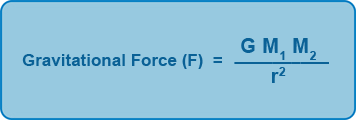## Gravitational Force, Centripetal Force & Orbits

The Sun, Earth, moon and all other objects in the solar system attract each other with a force called gravity. This gravitational force only attracts and never repels and can act over an infinite distance.
Newton explained this force in his law of universal gravitation. He stated that everybody in the universe attracts every other body with a force that is proportional to the product of the masses and inversely proportional to the square of the distance between them.

Mathematically this can be written as:Where M1 and M2 are two masses and r is the distance between them.

G is called the universal gravitational constant of gravitation as is equal to 6.67x10-11Nm2kg-2

The sun is the largest body in the solar system. It contains more than 99% of the mass of the solar system. The sun with it dominant mass exerts the greatest gravitational force in the solar system and holds all the other objects in orbit and governs their motion.

The planets travel around the sun in paths or orbits called ellipses. An ellipse is a slightly squashed circle. When an object moves in a circle its speed remains constant but as the direction is constantly changing so is its velocity. This is because velocity has size and direction whereas speed only has size. For an object moving in a circle a force is required to change the direction as defined by Newton’s first law of motion. This force constantly pulls the object towards the centre of the circle. A force that pulls an object towards the centre of a circle is called centripetal force. The source for the centripetal force in the solar system is the gravitational force of the sun. Without the centripetal force from the sun the planets would travel in a straight line. The velocity of the planets is high enough so that they continuously accelerate towards the sun without ever leaving their orbits. It is for this reason that the planets do not fall into the sun from its strong gravitational force of attraction.

### The Solar System and Orbits